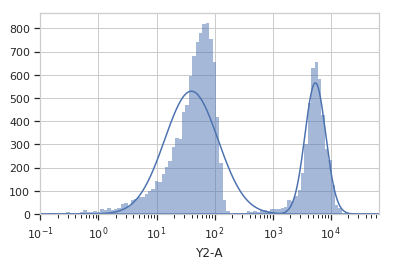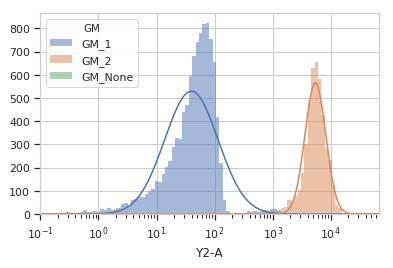# cytoflow.operations.gaussian_1d¶

class cytoflow.operations.gaussian_1d.GaussianMixture1DOp[source]

This module fits a Gaussian mixture model with a specified number of components to a channel.

Warning

GaussianMixture1DOp is DEPRECATED and will be removed in a future release. It doesn’t correctly handle the case where an event is present in more than one component. Please use GaussianMixtureOp instead!

Creates a new categorical metadata variable named name, with possible values name_1 …. name_n where n is the number of components. An event is assigned to name_i category if it falls within sigma standard deviations of the component’s mean. If that is true for multiple categories (or if sigma is 0.0), the event is assigned to the category with the highest posterior probability. If the event doesn’t fall into any category, it is assigned to name_None.

As a special case, if num_components is 1 and sigma > 0.0, then the new condition is boolean, True if the event fell in the gate and False otherwise.

Optionally, if posteriors is True, this module will also compute the posterior probability of each event in its assigned component, returning it in a new colunm named {Name}_Posterior.

Finally, the same mixture model (mean and standard deviation) may not be appropriate for every subset of the data. If this is the case, you can use the by attribute to specify metadata by which to aggregate the data before estimating (and applying) a mixture. The number of components is the same across each subset, though.

name

The operation name; determines the name of the new metadata column

Type: Str
channel

Which channel to apply the mixture model to.

Type: Str
num_components

How many components to fit to the data? Must be positive.

Type: Int (default = 1)
sigma

How many standard deviations on either side of the mean to include in each category? If an event is in multiple components, assign it to the component with the highest posterior probability. If sigma == 0.0, categorize all the data by assigning each event to the component with the highest posterior probability. Must be >= 0.0.

Type: Float (default = 0.0)
by

A list of metadata attributes to aggregate the data before estimating the model. For example, if the experiment has two pieces of metadata, Time and Dox, setting by = [“Time”, “Dox”] will fit the model separately to each subset of the data with a unique combination of Time and Dox.

Type: List(Str)
scale

Re-scale the data before fitting the model?

Type: Enum(“linear”, “log”, “logicle”) (default = “linear”)
posteriors

If True, add a column named {Name}_Posterior giving the posterior probability that the event is in the component to which it was assigned. Useful for filtering out low-probability events.

Type: Bool (default = False)

Examples

Make a little data set.

>>> import cytoflow as flow
>>> import_op = flow.ImportOp()
>>> import_op.tubes = [flow.Tube(file = "Plate01/RFP_Well_A3.fcs",
...                              conditions = {'Dox' : 10.0}),
...                    flow.Tube(file = "Plate01/CFP_Well_A4.fcs",
...                              conditions = {'Dox' : 1.0})]
>>> import_op.conditions = {'Dox' : 'float'}
>>> ex = import_op.apply()


Create and parameterize the operation.

>>> gm_op = flow.GaussianMixture1DOp(name = 'GM',
...                                  channel = 'Y2-A',
...                                  scale = 'log',
...                                  num_components = 2)


Estimate the clusters

>>> gm_op.estimate(ex)


Plot a diagnostic view

>>> gm_op.default_view().plot(ex)Apply the gate

>>> ex2 = gm_op.apply(ex)


Plot a diagnostic view with the event assignments

>>> gm_op.default_view().plot(ex2)estimate(experiment, subset=None)[source]

Estimate the Gaussian mixture model parameters.

Parameters: experiment (Experiment) – The data to use to estimate the mixture parameters subset (str (default = None)) – If set, a Python expression to determine the subset of the data to use to in the estimation.
apply(experiment)[source]

Assigns new metadata to events using the mixture model estimated in estimate().

Returns: A new Experiment, with a new column named name, and possibly one named name _Posterior. Also the following new statistics: mean : Float the mean of the fitted gaussian stdev : Float the inverse-scaled standard deviation of the fitted gaussian. on a linear scale, this is in the same units as the mean; on a log scale, this is a scalar multiple; and on a logicle scale, this is probably meaningless! interval : (Float, Float) the inverse-scaled (mean - stdev, mean + stdev) of the fitted gaussian. this is likely more meaningful than stdev, especially on the logicle scale. proportion : Float the proportion of events in each component of the mixture model. only set if num_components > 1. Experiment
default_view(**kwargs)[source]

Returns a diagnostic plot of the Gaussian mixture model.

Returns: IView an IView, call plot() to see the diagnostic plot.
class cytoflow.operations.gaussian_1d.GaussianMixture1DView[source]

A diagnostic view for a GaussianMixture1DOp.

facets

A read-only list of the conditions used to facet this view.

Type: List(String)
by

A read-only list of the conditions used to group this view’s data before plotting.

Type: List(String)
channel

The channel this view is viewing. If you created the view using default_view(), this is already set.

Type: String
scale

The way to scale the x axes. If you created the view using default_view(), this may be already set.

Type: {‘linear’, ‘log’, ‘logicle’}
op

The IOperation that this view is associated with. If you created the view using default_view(), this is already set.

Type: Instance(IOperation)
xfacet, yfacet

Set to one of the conditions in the Experiment, and a new row or column of subplots will be added for every unique value of that condition.

Type: String
huefacet

Set to one of the conditions in the in the Experiment, and a new color will be added to the plot for every unique value of that condition.

Type: String
huescale

How should the color scale for huefacet be scaled?

Type: {‘linear’, ‘log’, ‘logicle’}
plot(experiment, **kwargs)[source]

Plot the plots.

Parameters: experiment (Experiment) – The Experiment to plot using this view. title (str) – Set the plot title xlabel, ylabel (str) – Set the X and Y axis labels huelabel (str) – Set the label for the hue facet (in the legend) legend (bool) – Plot a legend for the color or hue facet? Defaults to True. sharex, sharey (bool) – If there are multiple subplots, should they share axes? Defaults to True. height (float) – The height of each row in inches. Default = 3.0 aspect (float) – The aspect ratio of each subplot. Default = 1.5 col_wrap (int) – If xfacet is set and yfacet is not set, you can “wrap” the subplots around so that they form a multi-row grid by setting col_wrap to the number of columns you want. sns_style ({“darkgrid”, “whitegrid”, “dark”, “white”, “ticks”}) – Which seaborn style to apply to the plot? Default is whitegrid. sns_context ({“paper”, “notebook”, “talk”, “poster”}) – Which seaborn context to use? Controls the scaling of plot elements such as tick labels and the legend. Default is talk. despine (Bool) – Remove the top and right axes from the plot? Default is True. min_quantile (float (>0.0 and <1.0, default = 0.001)) – Clip data that is less than this quantile. max_quantile (float (>0.0 and <1.0, default = 1.00)) – Clip data that is greater than this quantile. lim ((float, float)) – Set the range of the plot’s data axis. orientation ({‘vertical’, ‘horizontal’}) num_bins (int) – The number of bins to plot in the histogram. Clipped to [100, 1000] histtype ({‘stepfilled’, ‘step’, ‘bar’}) – The type of histogram to draw. stepfilled is the default, which is a line plot with a color filled under the curve. density (bool) – If True, re-scale the histogram to form a probability density function, so the area under the histogram is 1. Only seems to work if scale is linear. linewidth (float) – The width of the histogram line (in points) linestyle ([‘-‘ | ‘–’ | ‘-.’ | ‘:’ | “None”]) – The style of the line to plot alpha (float (default = 0.5)) – The alpha blending value, between 0 (transparent) and 1 (opaque). color (matplotlib color) – The color to plot the annotations. Overrides the default color cycle. plot_name (str) – If this IView can make multiple plots, plot_name is the name of the plot to make. Must be one of the values retrieved from enum_plots().
cytoflow.operations.gaussian_1d.poly_area(x, y)[source]